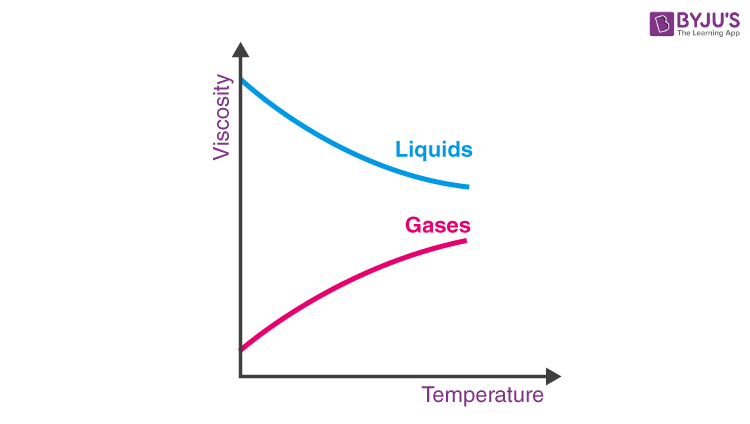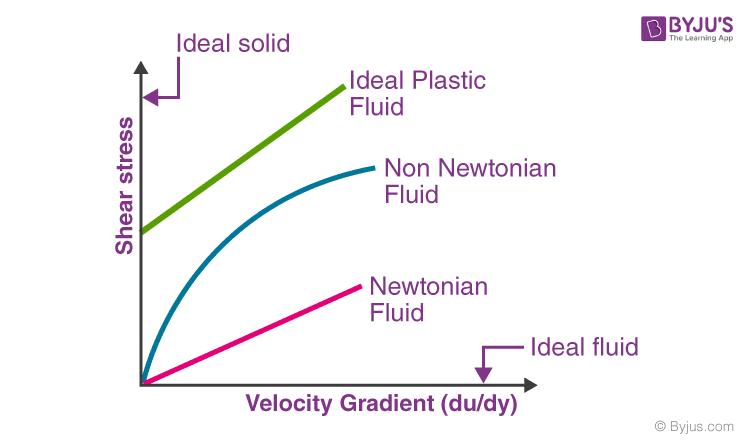# Fluid

We are familiar with solid, liquid, gas, and plasma states of matter. We also know how these matters behave when force or stress is applied to them. We have learned how the molecules in solid, liquid, and gas undergo change. In this session, let us learn in detail about fluids. Let us also know the types of fluids, characteristics of fluids, along with definitions and examples that relate to our daily life.

## What is a Fluid

Fluids undergo continuous deformation, i.e. fluids always keep flowing. To define fluid, it is a substance that does not possess a definite shape and easily yields to external pressure. Fluid is any liquid or gas or any material that is unable to withstand a shearing or tangential force, when at rest. When the said forces are applied to the fluid, it goes through a continuous change in shape. Fluids are substances with zero shear modulus, i.e they cannot resist the shear force applied to them.

In our day-to-today life, we would have heard the dehydrated patient advised to consume more fluids to compensate for the water loss. Fluids help in the digestion of food, hence we need to take more fluids.

### Classification of Fluids

Fluids are classified into four types depending on its flow, they are:

2. Compressible or incompressible
3. Viscous or non-viscous
4. Rotational or irrotational

Steady fluid: It is the fluid whose density remains constant at each and every point while flowing.

Unsteady fluid: It is the fluid whose velocity differs between any two points while flowing.

Compressible fluid and incompressible fluids: These are classified based on the mach number. The incompressible fluid has mach number<0.3 and the compressible fluid has mach number between 0.3 and 1.

Viscous or Non-viscous

Fluids with more thickness or viscosity are known as viscous fluids, they are generally very gloppy fluids. Examples: shampoo and motor oil.

Fluids with comparatively less thickness or viscosity are known as non-viscous fluids. These are the fluids with no resistance or negligible resistance to internal friction. Non-viscous fluids flow without any loss of kinetic energy.

Example: Superfluid liquid helium

Rotational or irrotational

If the angle between the two intersecting lines of the boundary of the fluid element changes while moving in the flow, the flow is a rotational flow. Depending on the angular motion of the fluid, it is classified into rotational fluid or irrotational fluid.

If the fluid rotates as a whole with no change in angles between the boundary lines, the flow of the fluid is classified as irrotational flow.

Based on the study of fluids, they are classified into two types, they are:

Fluids statics: It is the mechanism of fluids at rest or non-motion, and the pressure in fluids exerted by fluids on anybody.

Fluids dynamics – It involves the study of the flow of fluids at motion. Popular branches like aerodynamics and hydrodynamics are part of fluid dynamics.

### Properties of Fluids

After understanding what is fluid and its classification, let us know some of the main properties of fluids. Properties of fluids are:

1. Density

2. Viscosity

3. Temperature

4. Pressure

5. Specific Volume

6. Specific Weight

7. Specific Gravity

8. Surface Tension

9. Vapour Pressure

10. Capillarity

11. Cavitation

Density

Density is defined as the mass per unit volume of a fluid. The density of a substance varies as per the volume of a substance. The SI unit of density is

$$\begin{array}{l}kg.m^{-3}\end{array}$$

$$\begin{array}{l}\rho =\frac{mass of fluid}{volume of fluid}\end{array}$$

Viscosity

Viscosity determines the amount of resistance offered by the fluid to shear stress. A fluid that offers no resistance to shear stress is called an inviscid fluid.Temperature

The degree of hotness or coldness of the body is determined by the temperature. The temperature of the fluid is measured using Kelvin or Celsius or Fahrenheit. According to the International System of Units, the Kelvin scale is used.

Pressure

The pressure of a fluid is the force applied by it per unit area. Fluid pressure takes place in two conditions: open condition and closed condition. The pressure at any given point of a static fluid is called hydrostatic pressure.

$$\begin{array}{l}Pressure of the fluid = \frac{Force}{Area}\end{array}$$

Specific Volume

It is defined as the ratio of the volume of the material to its mass. Specific volume is the reciprocal of the density of the material. Specific volume is inversely proportional to density.

The SI unit of specific volume is

$$\begin{array}{l}m^{3}.kg^{-1}\end{array}$$

Specific Weight

The specific weight (γ) of a fluid is defined as the weight per unit volume. The SI unit of specific weight is

$$\begin{array}{l}N/m^{3}\end{array}$$
.

Specific weight is related to density as γ = ρg. The specific weight of fluid varies with temperature.

Specific Gravity

It is the ratio of the specific weight of the given fluid to the specific weight of standard fluid. Specific Gravity is also known as relative density. It is represented by the letter ‘S’ and has no unit.

Surface Tension

It is the tendency of liquid surfaces to shrink in minimum surface area. Surface tension is caused by cohesive forces between the liquid molecules.

Note: Surface tension is the main reason for most insects to float on the water despite being denser than water.

Vapour Pressure

The vapour pressure of a liquid is the equilibrium pressure of a vapour above its liquid. The vapour pressure of a liquid varies with temperature. As the temperature increases, the vapour pressure also increases.

Cavitation

The process of cavitation happens when bubbles or voids form within a fluid. This process takes place when the pressure drops instantaneously below the vapour pressure.

After knowing the properties of fluids, let us know various types of fluids.

## Types of Fluids

After learning the properties of fluids along with its classification, let us learn the types of fluids.

• Ideal fluid
• Real fluid
• Newtonian fluid
• Non-Newtonian fluid
• Ideal plastic fluid
• Incompressible fluid
• Compressible fluid

Ideal fluid: It is a fluid that does not have viscosity and cannot be compressed. This type of fluid cannot exist practically.

Real fluid: All types of fluids that possess viscosity are classified as real fluids. Examples: Kerosene and castor oil.

Newtonian fluid: A real fluid that abides by Newton’s law of viscosity is known as a Newtonian fluid. Example: Hydrogen and water

Non-Newtonian fluid: Fluids that do not abide by Newton’s law of viscosity are known as Non-Newtonian fluid. Example: Oobleck and flubber

Ideal plastic fluid: If the shear stress is directly proportional to the velocity gradient, and if the value of shear stress is greater than the resultant, it is referred to as ideal plastic fluid.

Incompressible fluid: If fluid’s density does not vary on the application of force, it is known as an incompressible fluid. Example: The stream of water flowing at high speed from a garden hose pipe.

Compressible fluid: If fluid’s density varies on the application of force, it is called a compressible fluid. Example: gas, vapour, and steam.## Frequently Asked Questions – FAQs

### What is a fluid?

A substance that does not possess a definite shape and easily yields to external pressure is known as a fluid.

### The study of the flow of fluids at motion is known as?

The study of the flow of fluids at motion is known as fluid dynamics.